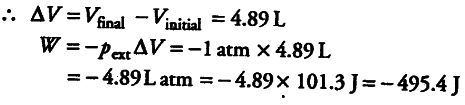# Calculate the work done when 11.2 g of iron dissolves

Calculate the work done when 11.2 g of iron dissolves in hydrochloric acid in
(i) a closed vessel
(ii) an open beaker at 25°C (Atomic mass of Fe = 56 u)

Iron reacts with HCI acid to produce { H }_{ 2 } gas as
Fe+ 2Hcl ------> { Fecl }_{ 2 } + { H }_{ 2 }

Thus, 1 mole of Fe, i.e., 56 g Fe produces { H }_{ 2 } gas = 1 mol.
11.2 g Fe will produce { H }_{ 2 } gas = 1/56 x 11.2 = 0.2 mol
(i) If the reaction is carried out in closed vessel, ∆V = 0
W = { -p }_{ ext } ∆V = 0
(ii) If the reaction is carried out in open beaker (external pressure being 1 atm)
Initial volume = 0 (because no gas is present)
Final volume occupied by 0.2 mole of { H }_{ 2 } at 25°C and 1 atm pressure can be calculated as follows pV = nRT
V = nrt/p
= 0.2 mol x 0.0821 L atm/K/mol x 298K / 1 atm
=4.89 L